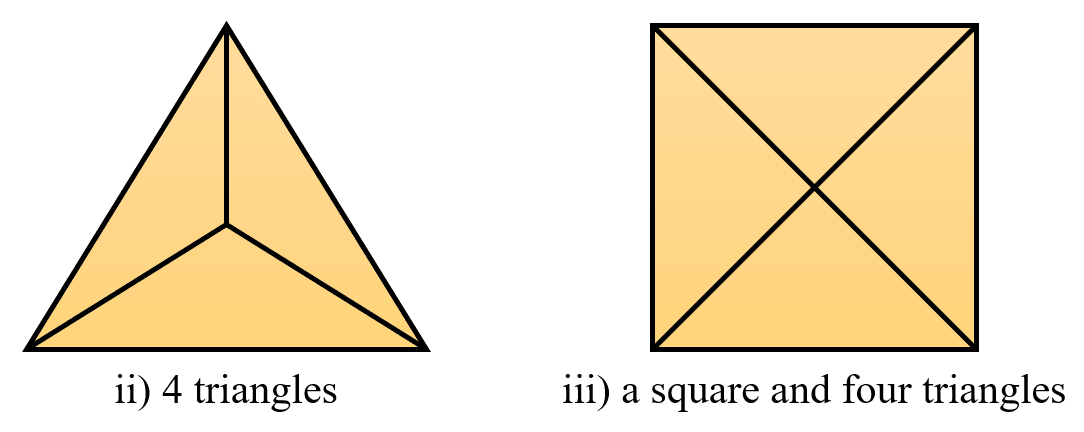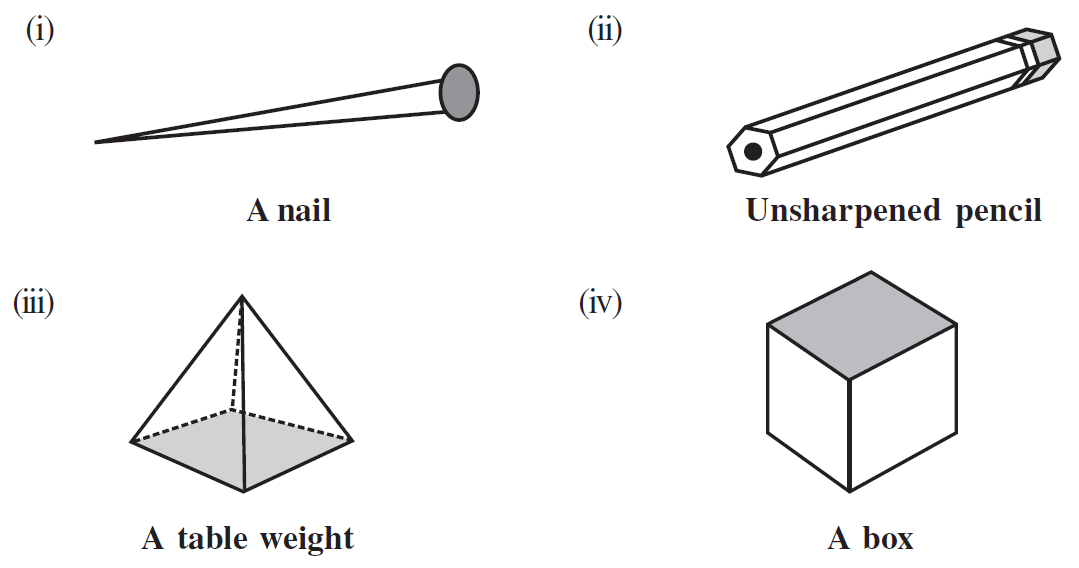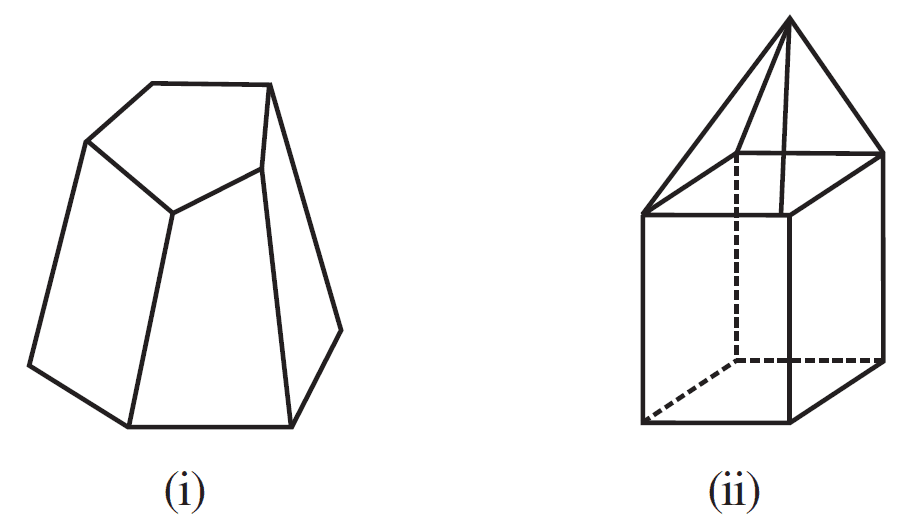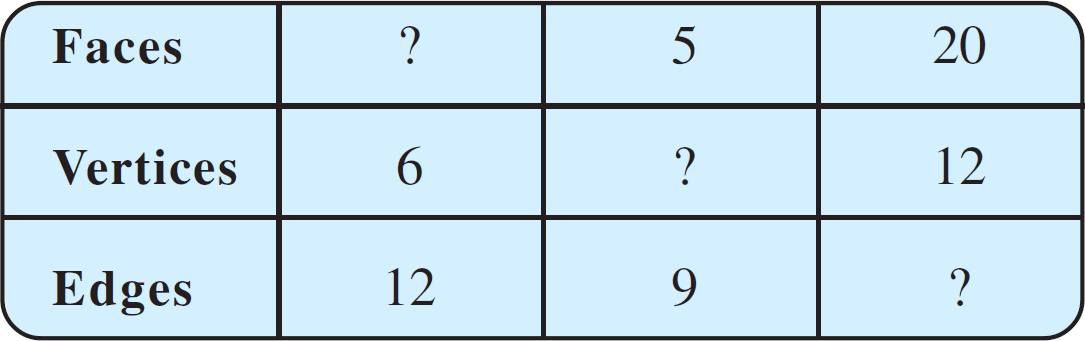# vs.eyeandcontacts.com

## Chapter 10 Visualising Solid Shapes Exercise 10.3

Question 1: Can a polyhedron have for its faces
i) 3 triangles?
ii) 4 triangles?
iii) a square and four triangles?

i) No, a polyhedron can’t have 3 triangles for its faces because polyhedron must have edges meeting at the vertices called points.
ii) Yes, a polyhedron can have 4 triangles for its faces because all the edges are meeting at the vertices called points.
iii) Yes, a polyhedron can have a square and four triangles for its faces because all the edges are meeting at the vertices called points.Question 2: Is it possible to have a polyhedron with any given number of faces? (Hint: Think of a pyramid).
Yes, it is possible to have a polyhedron with the number of faces is greater than or equal to 4. Example: Pyramid with 4 faces.

Question 3: Which are prisms among the following?Answer: ii) Unsharpened pencil and iii) A table weight are prisms.

Question 4:
i) How are prisms and cylinders alike?
ii) How are pyramids and cones alike?

i) A prism becomes a cylinder as the number of sides of its base becomes larger and larger.
ii) A pyramid becomes a cone as the number of sides of its base becomes larger and larger.

Question 5: Is a square prism same as a cube? Explain.
No, every square prism is not same as cube. It may be a cuboid also.

Question 6: Verify Euler’s formula for these solids.i)
Number of faces = 7
Number of edges = 15
Number of vertices = 10
by using Euler’s formula
= F + V - E = 2
= 7 + 10 - 15 = 2
= 17 - 15 = 2
= 2 = 2
Hence, verified.

ii)
Number of faces = 9
Number of edges = 16
Number of vertices = 9
by using Euler’s formula
= F + V - E = 2
= 9 + 9 - 16 = 2
= 18 - 16 = 2
= 2 = 2
Hence, verified.

Question 7: Using Euler’s formula find the unknown.i)
Number of faces = x
Number of vertices = 6
Number of edges = 12
by using Euler’s formula
= F + V - E = 2
= x + 6 - 12 = 2
= x - 6 = 2
= x = 6 + 2
= x = 8
Therefore, the number of the faces of the solid is 8.

ii)
Number of faces = 5
Number of vertices = x
Number of edges = 9
by using Euler’s formula
= F + V - E = 2
= 5 + x - 9 = 2
= 5 + x = 2 + 9
= 5 + x = 11
= x = 11 - 5
= x = 6
Therefore, the number of the vertices of the solid is 6.

iii)
Number of faces = 20
Number of vertices = 12
Number of edges = x
by using Euler’s formula
= F + V - E = 2
= 20 + 12 - x = 2
= 32 - x = 2
= 32 - 2 = x
= 30 = x
Therefore, the number of the edges of the solid is 30.

Question 8: Can a polyhedron have 10 faces, 20 edges and 15 vertices?

Number of faces = 10
Number of vertices = 15
Number of edges = 20
by using Euler’s formula
= F + V - E = 2
= 10 + 15 - 20 = 2
= 25 - 20 = 2
= 5 ≠ x
Therefore, a polyhedron can’t have 10 faces, 20 edges and 15 vertices.